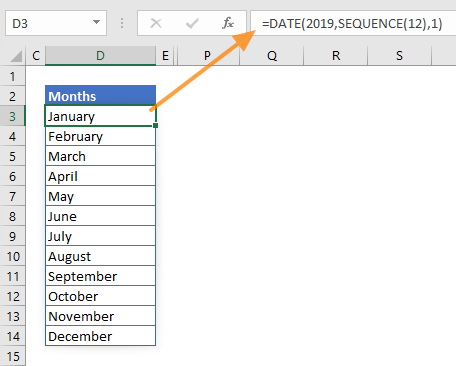Date lists are an important part of numerous Excel models. Excel can automatically recognize date-time values, format the cells, and generate a date list quickly with AutoFill or specific functions. However, date-time functions are focused on a generating a single input, just like most other Excel functions. In September 2018, Microsoft has introduced a new the concept of dynamic arrays and “spill” behavior to overcome this limitation.

The SEQUENCE function also came with this update. This function essentially generates an array of a number sequence. For example, you can generate numbers from 1 to 10 that increase by 1 at each step. Let's see how the SEQUENCE function can help generate a date list dynamically.

At the time of writing this article, Microsoft announced that this feature is currently only available to a number of select users. When it’s ready, the feature is planned to be released for Office 365 users.

## SEQUENCE Function Basics

The function can generate an array of sequential numbers. You can define how many rows or columns of numbers are to be generated, the start value, and the increment of each step.

=SEQUENCE(rows,[columns],[start],[step])

 rows The number of rows to be returned. [columns] Optional. The number of columns to be returned. The default value is 1. [start] Optional. Starting value. The default value is 1. [step] Optional. The increment of each step between values. The default value is 1.

## Date-time values in Excel

Excel keeps date and time values as numbers. Excel assumes that Jan 1st, 1900 is 1, and every subsequent date value is based on this. While whole numbers represent days, decimals represent time values. For example; 1/1/2018 is equal to 43101, and 12:00 is equal to 0.5. As a result, adding or subtracting a number from a date value means adding or removing days from a date value.

## Generating a list of days

In Excel, days are essentially numbers. All we need to do is format the number that returns the SEQUENCE function to generate the date list. However, without setting a [start] value, the SEQUENCE function starts from 1. For this, you can use a cell that already has a date value or a formula that returns a date value like TODAY(), NOW() or DATE().

We used the TODAY function in our example with 7 rows and a single column to generate 7 days starting from today (the date this article was written).

=SEQUENCE(7,1,TODAY())

After entering the formula, the dates will be generated automatically. Remember to format generated values to display them as dates instead of numbers.## Generating a list of months

Generating a months list work in a similar way. However, since months have different day counts, generating dates by constant increments won't work.

The DATE function has 3 arguments, the second being month. If you set 1 for  month, the DATE function generates a date on January. As a result, if you enter an array of numbers from 1 to 12, you will get dates from January to December.

=DATE(2019,SEQUENCE(12),1)

The formula generates first day of each month in 2019. Apply custom number formatting "mmmm" to show only the month names.## Generating a list of years

Generating years works similarly to months. The only difference is that you need set a [start] value to avoid starting the years from the 1900s. Before setting the [start] argument, you also need to set [columns]. Here, we set this to 1 to generate a list in a single column. If you want to generate a list through columns, set rows to 1.

=DATE(SEQUENCE(10,1,2019),1,1)

This formula will generate first days of 10 consecutive years starting from 2019.## Bonus: Calendar

You can also generate a calendar using the SEQUENCE function. By using both rows and [columns] arguments, you can make the DATE function spread through multiple rows and columns just like a calendar.

We start our calendar from April 1st. To generate only the days of a month, a 7-column, 5-row range is populated.

=SEQUENCE(5,7,DATE(2019,4,1))

Note: Here, we also used conditional formatting to hide the days in May. The formula is =MONTH(H5)<>4.